## 6.2. Calculate Cpk

Cpk takes into account the center of the data relative to the specifications, as well as the variation in the process. Cpk is simple to calculate. The smaller of the two Z values is selected. This is known as Zmin . When Zmin has been selected, it is divided by 3. The formula is: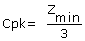The Z values for the example are Zupper of 2.00 and Zlower of 5.00, therefore Zmin is 2.00. Cpk for the example is: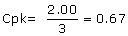If the Cpk formula is written in full, it becomes more apparent how Cpk works.This is the smaller of: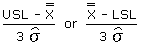Graphically this can be drawn for the example as follows: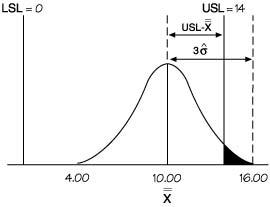The diagram clearly shows that the overall average is too close to the upper specification. By taking the smaller of the two Z values, Cpk is always looking at the worst side, where the specification is closest to the overall average. Since it is looking only at half the picture, instead of dividing by 6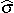as in Cp, it is divided by 3.

A Cpk value of one indicates that the tail of the distribution and the specification are an equal distance from the overall average, as shown below: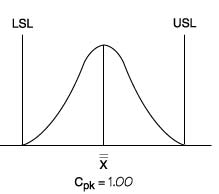A Cpk of less than one, as in the example, means that some of the data is beyond the specification limit. A Cpk greater than one indicates that the data is within the specification. The larger the Cpk, the more central and within specification the data.

The above article is an excerpt from the “Operational definition” chapter of Practical Tools for Continuous Improvement Volume 2 Statistical Tools.